Examples

Chapter 5 Class 11 Complex Numbers
Serial order wise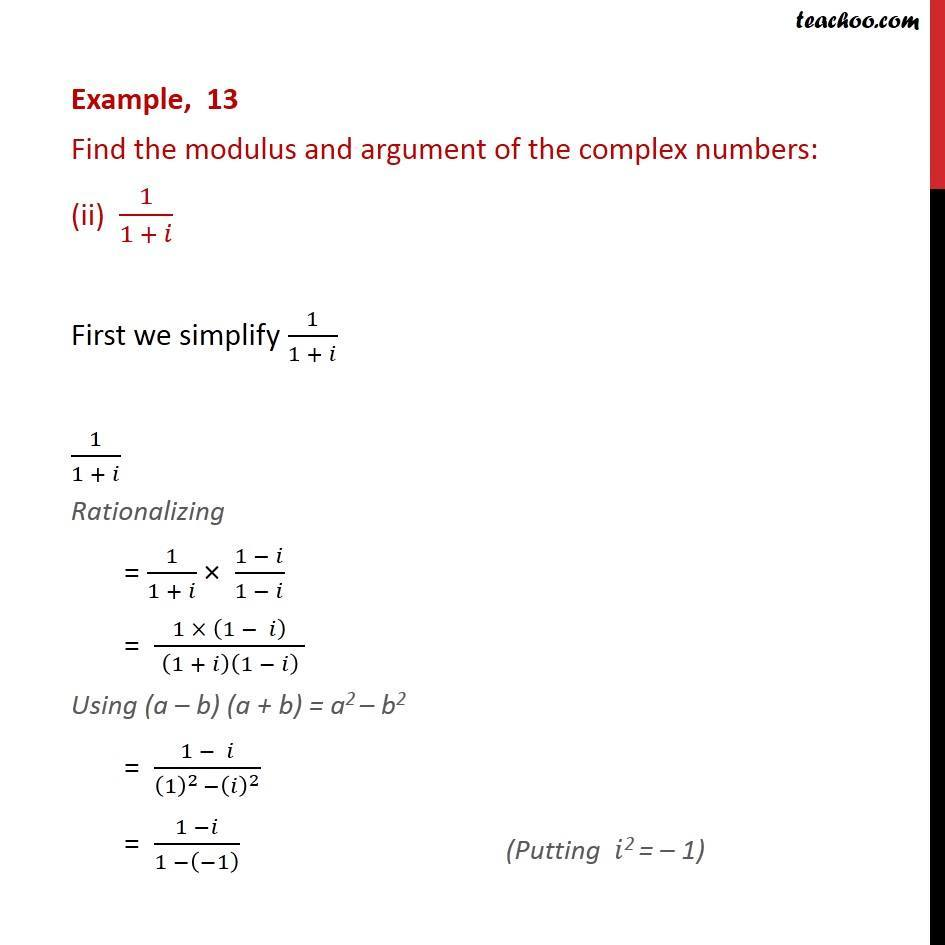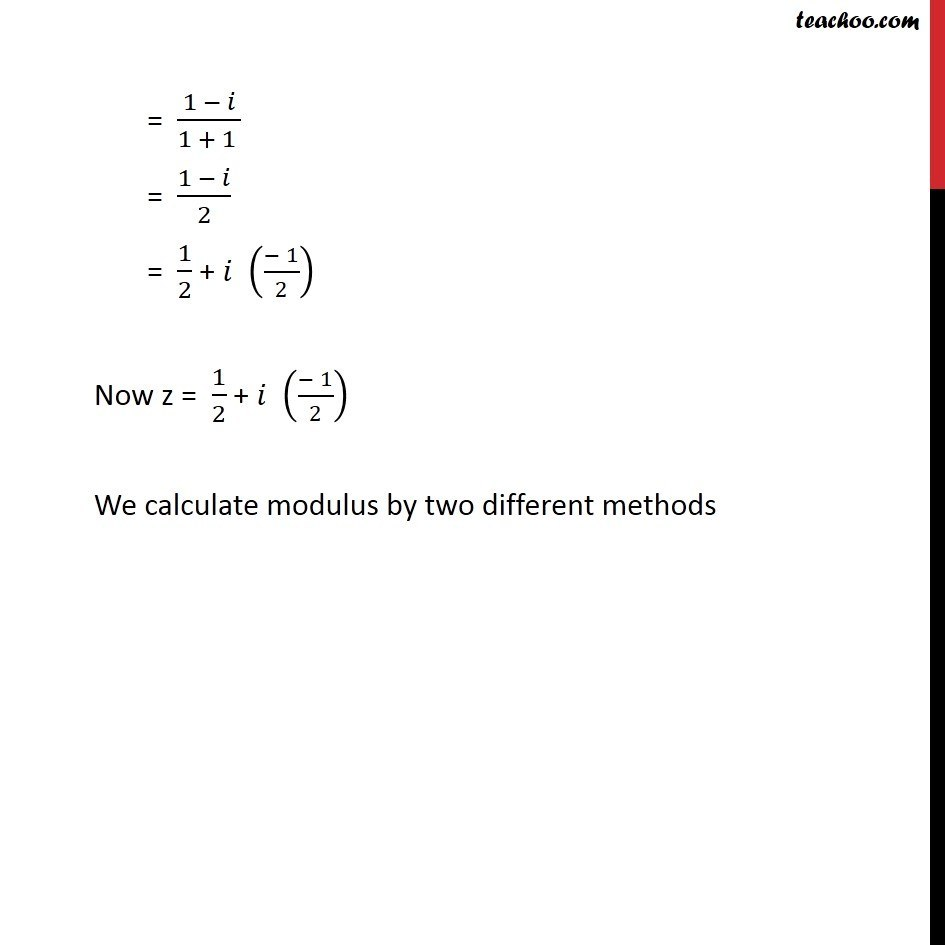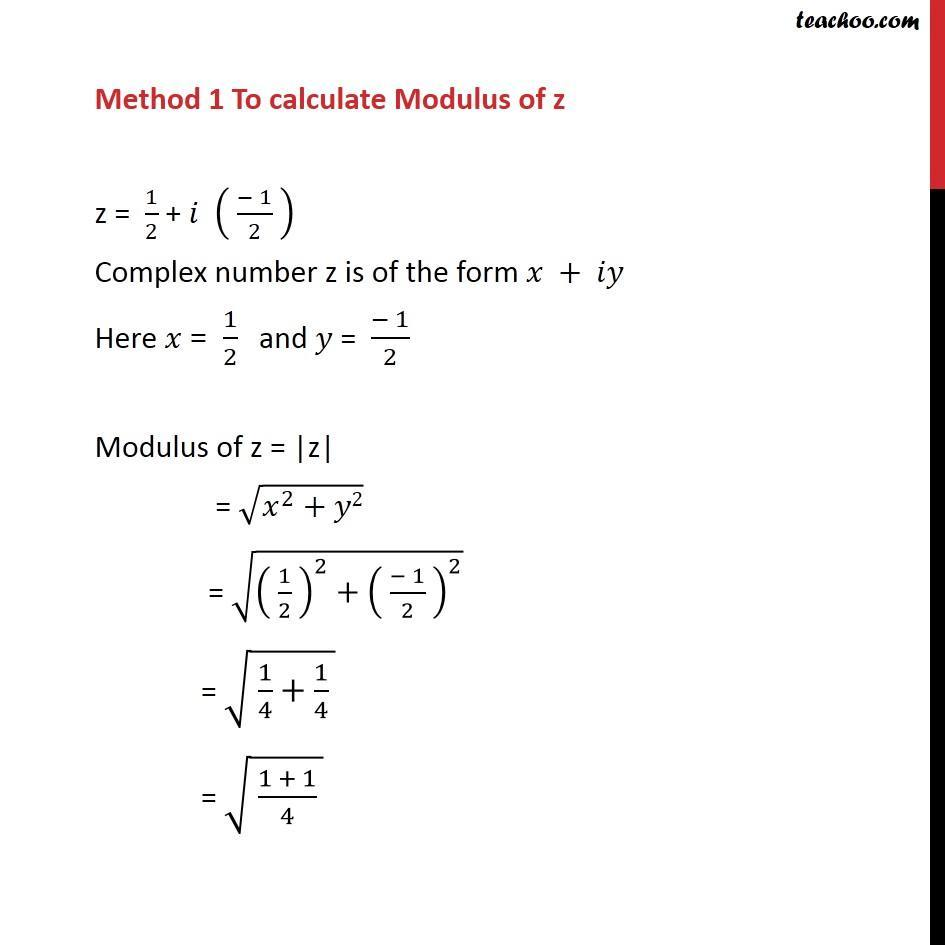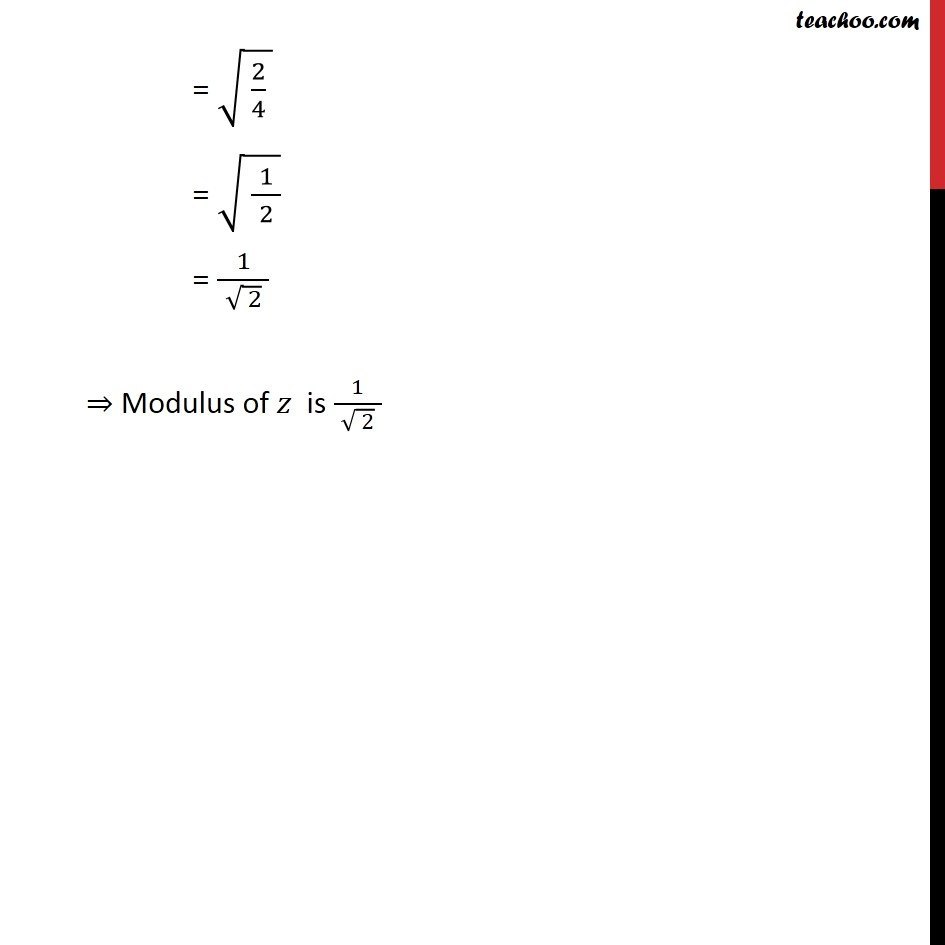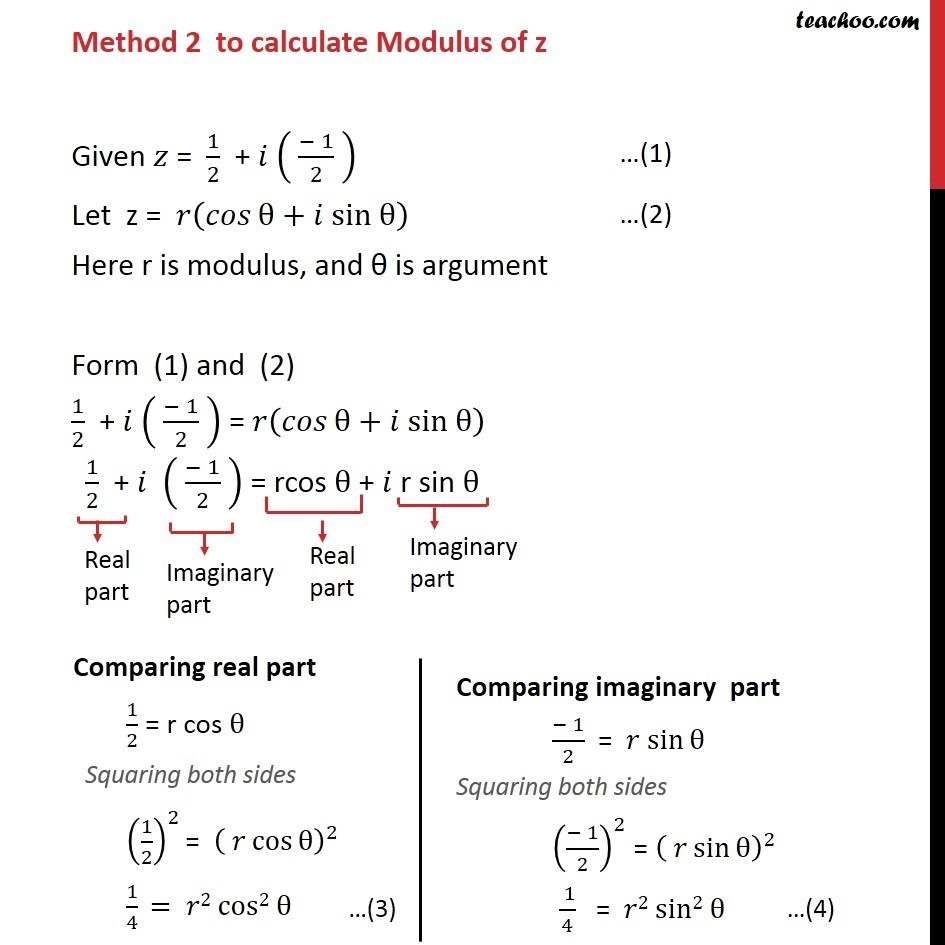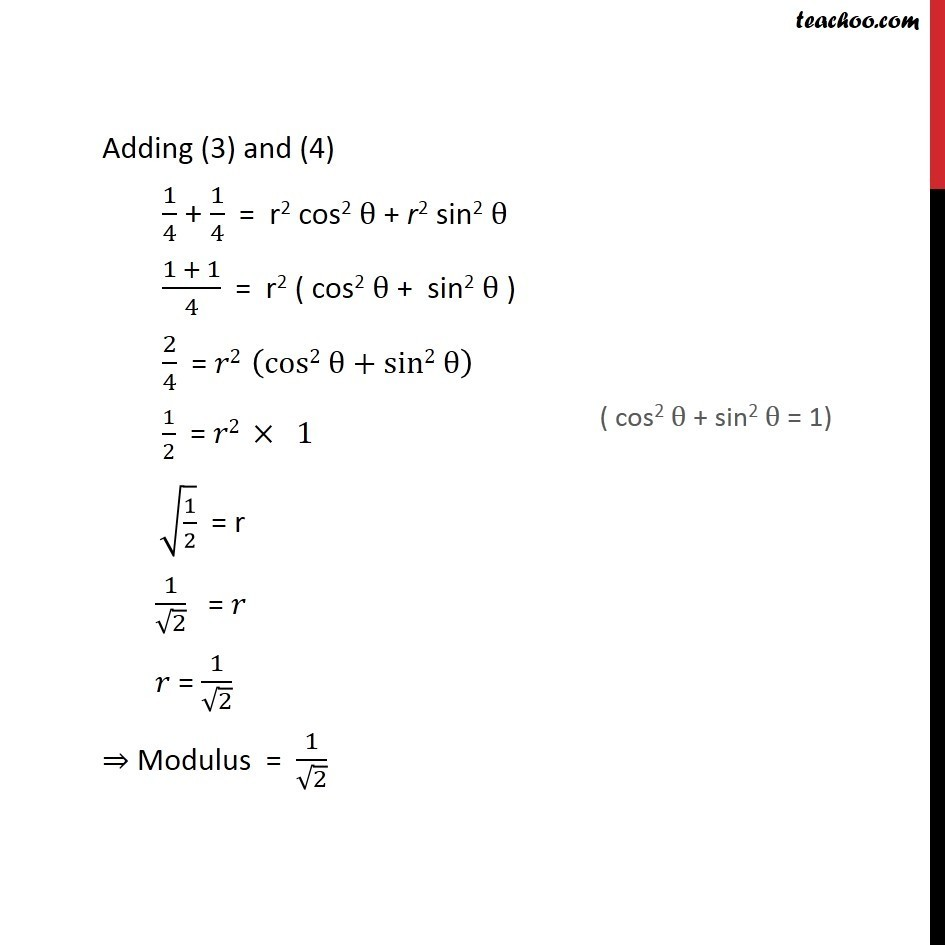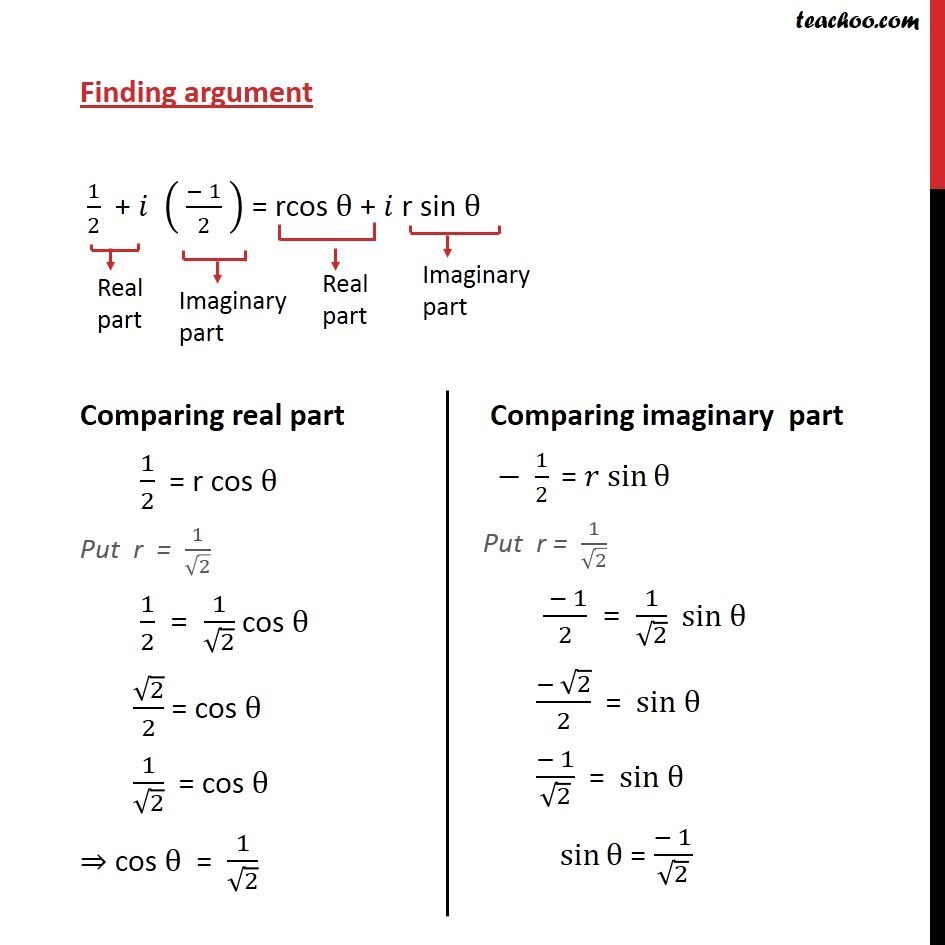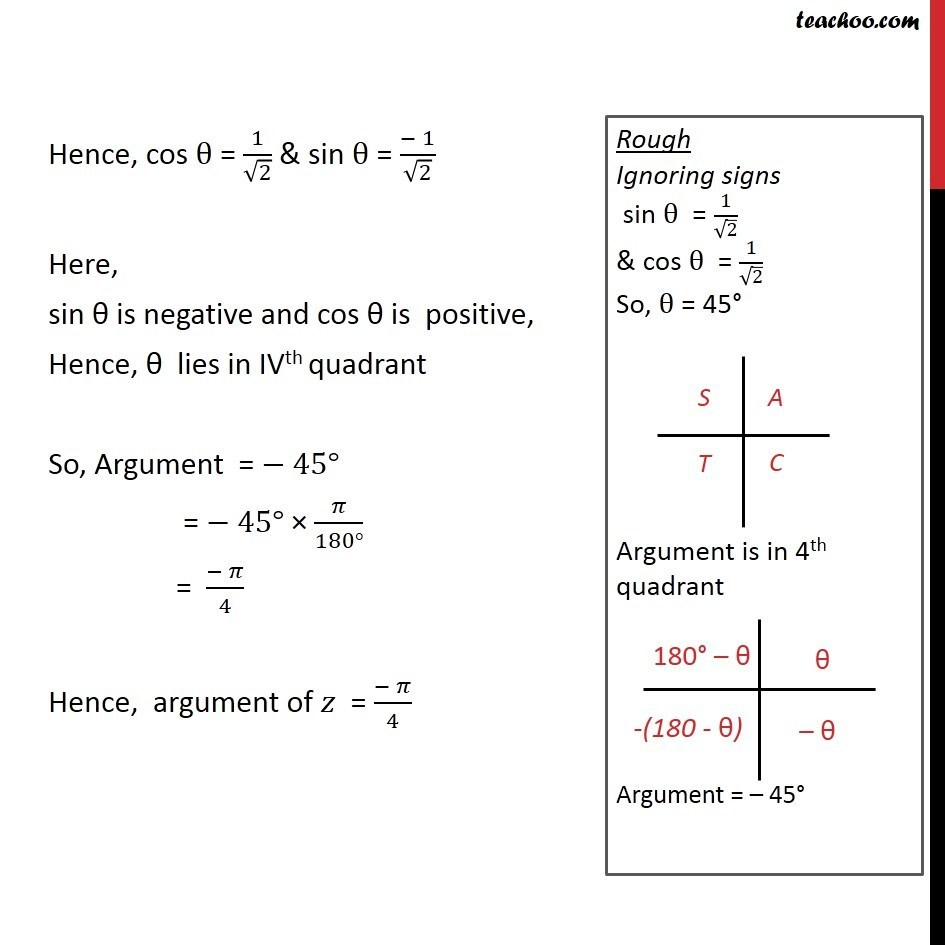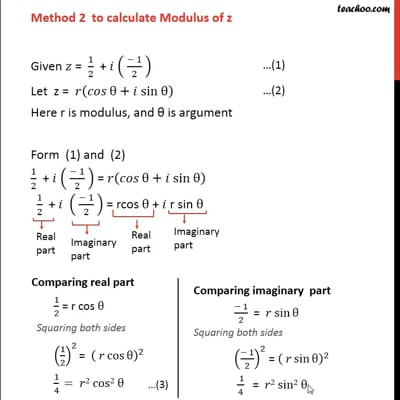This video is only available for Teachoo black users

Maths Crash Course - Live lectures + all videos + Real time Doubt solving!

### Transcript

Example, 13 Find the modulus and argument of the complex numbers: (ii) 1/(1 + 𝑖) First we simplify 1/(1 + 𝑖) 1/(1 + 𝑖) Rationalizing = 1/(1 + 𝑖) × (1 − 𝑖)/(1 − 𝑖) = (1 × (1 − 𝑖))/(" " (1 + 𝑖)(1 − 𝑖) ) Using (a – b) (a + b) = a2 – b2 = (1 − 𝑖)/((1)^2 −(𝑖)^2 ) = (1 −𝑖)/(1 −(−1) ) = (1 − 𝑖)/(1 + 1 ) = (1 − 𝑖)/2 = 1/2 + 𝑖 ((− 1)/2) Now z = 1/2 + 𝑖 ((− 1)/2) We calculate modulus by two different methods Method 1 To calculate Modulus of z z = 1/2 + 𝑖 ( (− 1)/2 ) Complex number z is of the form 𝑥 + 𝑖𝑦 Here 𝑥 = 1/2 and 𝑦 = (− 1)/2 Modulus of z = |z| = √(𝑥^2+𝑦2) = √(( 1/2 )^2+( (− 1)/2 )^2 ) = √( 1/4+1/4 ) = √( (1 + 1)/4 ) = √( 2/4 ) = √( 1/(" " 2" " )) = 1/(" " √( 2) " " ) ⇒ Modulus of 𝑧 is 1/(" " √( 2) " " ) Method 2 to calculate Modulus of z Given 𝑧 = 1/2 + 𝑖 ( (− 1)/2 ) Let z = 𝑟(𝑐𝑜𝑠⁡θ+𝑖 sin θ) Here r is modulus, and θ is argument Form (1) and (2) 1/2 + 𝑖 ( (− 1)/2 ) = 𝑟(𝑐𝑜𝑠⁡θ+𝑖 sin θ) 1/2 + 𝑖 ( (− 1)/2 ) = rcos θ + 𝑖 r sin θ Adding (3) and (4) 1/4 + 1/4 = r2 cos2 θ + r2 sin2 θ (1 + 1)/4 = r2 ( cos2 θ + sin2 θ ) 2/4 = 𝑟2 (cos2 θ+sin2 θ) 1/2 = 𝑟2 × 1 √(1/2) = r 1/√2 = 𝑟 𝑟 = 1/√2 ⇒ Modulus = 1/√2 Finding argument 1/2 + 𝑖 ( (− 1)/2 ) = rcos θ + 𝑖 r sin θ Comparing real part 1/2 = r cos θ Put r = 1/√2 1/2 = 1/√2 cos θ √2/2 = cos θ 1/√2 = cos θ ⇒ cos θ = 1/√2 Hence, cos θ = 1/√2 & sin θ = (− 1)/√2 Here, sin θ is negative and cos θ is positive, Hence, θ lies in IVth quadrant So, Argument = − 45° = − 45° × 𝜋/(180°) = (− 𝜋)/4 Hence, argument of 𝑧 = (− 𝜋)/4